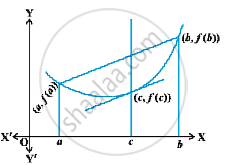# Mean Value Theorem

#### theorem

(Rolle’s Theorem)
Let f : [a, b] → R be continuous on [a, b] and differentiable on (a, b), such that f(a) = f(b), where a and b are some real numbers. Then there exists some c in (a, b) such that f′(c) = 0.
In following  fig. graphs of a few typical differentiable functions satisfying the hypothesis of Rolle’s theorem are given.The slope of the tangent to the curve at various points between a and b. In each of the graphs, the slope becomes zero at least at one point. That is precisely the claim of the Rolle’s theorem as the slope of the tangent at any point on the graph of y = f(x) is nothing but the derivative of f(x) at that point.

#### theorem

(Mean Value Theorem)
Let f : [a, b] → R be a continuous function on [a, b] and differentiable on (a, b). Then there exists some c in (a, b) such that
f'(c) = (f(b) - f(a))/ (b-a)
Observe that the Mean Value Theorem (MVT) is an extension of Rolle’s theorem. Let us now understand a geometric interpretation of the MVT. The graph of a function y = f(x) is given in the Fig.We have already interpreted f′(c) as the slope of the
tangent to the curve y = f(x) at (c, f(c)). in above fig. it is clear that
(f(b)-f(a))/(b-a)  is the slope of the secant drawn between (a, f(a)) and (b, f(b)).  The MVT states that there is a point c in (a, b) such that the slope of the tangent at (c, f(c)) is same as the slope of the secant between (a, f(a)) and (b, f(b)).
In other words, there is a point c in (a, b) such that the tangent at (c, f(c)) is parallel to the secant between (a, f(a)) and (b, f(b)).

#### description

• Rolle’s and Lagrange’s Mean Value Theorems (without proof) and their geometric interpretations
If you would like to contribute notes or other learning material, please submit them using the button below.

#### Video Tutorials

We have provided more than 1 series of video tutorials for some topics to help you get a better understanding of the topic.

Series 1

Series 2

Series 3

### Shaalaa.com

Rolle's Theorem and Lagrange's Mean Value Theorem [00:24:00]
S
0%